• 文章目录朴素贝叶斯基本原理为什么后验概率在最大化实例—学习一个贝叶斯分类器拉普拉斯修正优缺点 朴素贝叶斯基本原理 朴素贝叶斯(navie Bayes) 是基于贝叶斯定理与特征条件独立假设的分类方法。 先来了解一下这两...
文章目录朴素贝叶斯基本原理为什么后验概率在最大化实例—学习一个贝叶斯分类器拉普拉斯修正优缺点
朴素贝叶斯基本原理
朴素贝叶斯(navie Bayes) 是基于贝叶斯定理与特征条件独立假设的分类方法。
先来了解一下这两个基本概念。
（1）贝叶斯定理：
$P(y_i|x) = \frac{P(x,y_i)}{P(x)} = \frac{P(y_i) P(x|y_i)}{P(x)} = \frac{P(y_i) P(x|y_i)}{\sum_{j=1}^kP(y_j)P(x|y_j)}$
其中：$p(y)$ 为先验概率，
​		   $p(x|y)$ 称为似然，
​			$p(y|x)$ 称为后验概率
（2）条件独立假设
$P(X=x|Y=c_k)=P(X^{(1)},\dots,X^{(n)}|Y=c_k)\ =\prod^n_{j=1}P(X^{(j)}=x^{(j)}|Y=c_k)$
条件独立假设这里给定$Y$的情况下：每一个$X_i$和其他的每个$X_k$是条件独立的。
为了更好的说明朴素贝叶斯的基本原理，我们声明一些基本符号：
$\vec X$是定义在输入空间上的随机向量，$\vec x$ 是具体的向量，$\vec X = \vec x = (x^1,x^2,\ldots x^d)$ 表示一西瓜的属性 ，如色泽，纹理，触感等d个不同的属性；$Y$是定义在输出空间上的随机变量,$y$ 是具体的取值，$y \in \{c_1,c_2,\ldots c_k\}$ ，是数据的标签，如西瓜是好瓜还是坏瓜；$P(\vec X,Y)$ 是$\vec X$和$Y$的联合分布。有训练数据集如下：
$T = \{(\vec x_1, y_1),(\vec x_2, y_2)\ldots,(\vec x_n, y_n) \}$
注：我们规定 $x_i^{(j)}$ 表示第 $i$个样本的 第 $j$个属性
我们希望通过西瓜的一些基本属性向量$\vec X$ 对西瓜进行分类，判断西瓜是好瓜还是坏瓜，一个简单的二分类问题。接下来我们要介绍朴素贝叶斯是如何做到的。
第一步：朴素贝叶斯先学习先验概率分布：
假设样本独立同分布，则有：
$P(Y=c_k)=\frac{\sum_{i=1}^N I(y_i = c_k)}{N} , \quad k =1,2\ldots K$
其中$N$表示样本总数，当$y_i = c_k$ 时，$I(y_i = c_k)=1$ 。
如：在西瓜分类问题中，计算所有西瓜中好瓜的概率和坏瓜的概率。
$P(Y="好瓜") = \frac{好瓜的数目}{西瓜总数}$
第二步：学习条件(似然)分布：
$P(X=x|Y=c_k) = P(X^{1}=x^{1},X^{2}=x^{2}\ldots X^{n}=x^{n}|Y=c_k)$
那么，这里需要学习多少个概率值呢？如果每个特征 $x^{(j)}$ 有$S_j$ 个取值，$j = 1,2 \ldots n$ ，$Y$ 的取值有 $K$ 个，那么需要计算的概率数一共是 $K \prod^n_{j=1} S_j$ 个。
根据条件独立假设则有：
\begin{aligned} P(X=x|Y=c_k) &= P(X^{1}=x^{1},X^{2}=x^{2}\ldots X^{n}=x^{n}|Y=c_k) \\ &=\prod^n_{j=1}P(X^{(j)}=x^{(j)}|Y=c_k) \end{aligned}
引入条件独立假设，这里需要学习的概率值个数是 $K \sum^n_{j=1} S_j$ 个。
那么如何求解一个$P(X^{(j)}=x^{(j)}|Y=c_k)$呢？
假设第$j$ 个特征 $x^{(j)}$ 的取值集合为$\{a_{j1},a_{j2}\ldots,a_{jS_j} \}$，则有：
$P(X^{(j)}=a_{(jl)}|Y=c_k) = \frac{\sum_{i=1}^N I(x_i^{(j)}=a_{(jl)},y_i = c_k)}{\sum_{i=1}^N I(y_i = c_k)}$
$j = 1,2\ldots,n;l = 1,2\ldots,S_j;j = 1,2\ldots,K$
如，计算西瓜属性中色泽=“乌黑”这一特征的分布：
$P(色泽=“乌黑”|Y=“好瓜”) = \frac{既是好瓜同时又色泽乌黑的西瓜的个数}{好瓜总数}$
$P(色泽=“乌黑”|Y=“坏瓜”) = \frac{既是坏瓜同时又色泽乌黑的西瓜的个数}{坏瓜总数}$
条件独立假设即用于分类的特征在确定类的前提下都是独立的，这一假设使得朴素贝叶斯变得简单，但有时会牺牲一定的分类准确率。
第三步：计算后验分布$P(Y=c_k| \vec X = \vec x)$ :
计算后验根据贝叶斯定理进行：
\begin{aligned} P(Y=c_k| \vec X = \vec x) &=\frac{P(Y=c_k,\vec X = \vec x)}{p(\vec X = \vec x)} \\ &= \frac{P(Y=c_k)P(\vec X = \vec x|Y=c_k)}{\sum_{k=1}^K P(Y=c_k)P(\vec X = \vec x|Y=c_k)} \end{aligned}
结合第二步学习到的似然概率，则有：
\begin{aligned} P(Y=c_k| \vec X = \vec x) &= \frac{P(Y=c_k)P(\vec X = \vec x|Y=c_k)}{\sum_{k=1}^K P(Y=c_k)P(\vec X = \vec x|Y=c_k)} \\ \\ &= \frac{P(Y=c_k)\prod^n_{j=1}P(X^{(j)}=x^{(j)}|Y=c_k)}{\sum_{k=1}^K P(Y=c_k)\prod^n_{j=1}P(X^{(j)}=x^{(j)}|Y=c_k)} \end{aligned}
这就是朴素贝叶斯的基本公式。在好瓜与坏瓜的二分类任务中，我们可以计算，给定输入 $\vec x$ 我们可以计算好瓜的概率$P(Y=c_1| \vec X = \vec x)$，再计算坏瓜的概率$P(Y=c_2| \vec X = \vec x)$ 二者中大者为最终结果。由此，朴素贝叶斯的分类器可表示为：
$P(Y=c_k| \vec X = \vec x) = \arg \max\limits_{c_k} \frac{P(Y=c_k)\prod^n_{j=1}P(X^{(j)}=x^{(j)}|Y=c_k)}{\sum_{k=1}^K P(Y=c_k)\prod^n_{j=1}P(X^{(j)}=x^{(j)}|Y=c_k)}$
因为上式中的分母对所有的 $c_k$ 都是相同的，所以
$y = P(Y=c_k| \vec X = \vec x) = \arg \max\limits_{c_k} P(Y=c_k)\prod^n_{j=1}P(X^{(j)}=x^{(j)}|Y=c_k)$
总结一下以上步骤：说明以下两点：
(1) 似然概率 $P(X=x|Y=c_k)$ 可以理解为在给定类别 $Y = c_k$ 的前提下，分析当前类的数据具有什么样的性质特点，即朴素贝叶斯在对每一类数据的属性进行学习，形成一个个类的模板。
（2） 模板学习结束以后，后验概率上场 $P(Y=c_k| \vec X = \vec x)$，给定一个个样本的属性$\vec x$  ,然后到模板中去匹配，匹配度最高的为其类别。
为什么后验概率在最大化
那么，为什么匹配度最高的为其最终类别，也就是为什么后验概率在最大化者为其最终类别？ 我们从期望风险最小化的角度来详述。
我们假设选择0-1 损失作为损失函数：
$L(Y,f(\vec X)) = \left \{ \begin{array}{lr} 1, Y \neq f(\vec X) \\0 , Y = f(\vec X) \end{array} \right.$
$f(\vec X )$ 是分类决策函数。此时，期望风险函数(对损失函数求希望)为：
$R_{exp}(f) = E[L(Y,f(\vec X))]$
期望是对联合分布$P(\vec X, Y)$ 取的，所以条件期望为：
$R_{exp}(f) = E_X E_Y[L(c_k,f(\vec X))]P(c_k,\vec X)=E_X \sum_{k=1}^K[L(c_k,f(\vec X))]P(c_k|\vec X)$
$\sum_{k=1}^K[L(c_k,f(\vec X))]P(c_k|\vec X)$  为在$\vec X=\vec x$时的y的条件期望。
为了使期望风险最小化，只需对$\vec X = \vec x$  逐个极小化，所以有：
\begin{aligned} f(\vec x ) &= \arg \min_{y \in Y} \sum_{k=1}^KL(c_k;y)P(c_k|\vec X = \vec x) \\ &= \arg \min_{y \in Y} \sum_{k=1}^KP(y \neq c_k|\vec X = \vec x) \qquad 当y = c_k时,L(c_k;y)=0.\\ &=\arg \min_{y \in Y} (1-P(y \neq c_k|\vec X = \vec x) ) \\ &=\arg \max_{y \in Y}P(y=c_k|\vec X = \vec x) \end{aligned}
这就是后验概率最大化的准则。
实例—学习一个贝叶斯分类器
要求从如下数据集中学习一个分类器，并确定$x=(2,S)^T$ 的类别$y$ ,表中$X^{(1)},X^{(1) }$ 为特征，取值集合分别为 $A_1 = \{1,2,3\},A_2=\{S,M,L\}$ ，$Y$ 为类标记，$Y = \{1,-1\}$第一步：学习先验概率分布
$P(Y=1) = \frac{9}{15} \qquad P(Y=-1) = \frac{6}{15}$
第二步：学习条件(似然)分布：
计算之前，我们先考虑一共需要计算多少个值？
上文已经提过一共是 $K \sum^n_{j=1} S_j = 2\times(3+ 3)=12$ 个。
\begin{aligned} &P(X^{(1)} = 1|Y=1) = \frac{2}{9} \qquad P(X^{(1)} = 2|Y=1) = \frac{3}{9} \qquad P(X^{(1)} =3|Y=1) = \frac{4}{9} \\ &P(X^{(2)} = S|Y=1) = \frac{1}{9} \qquad P(X^{(2)} = M|Y=1) = \frac{2}{6} \qquad P(X^{(2)} =L|Y=1) = \frac{1}{6} \\ &P(X^{(1)} = 1|Y=-1) = \frac{3}{6} \qquad P(X^{(1)} = 2|Y=-1) = \frac{3}{6} \qquad P(X^{(1)} =3|Y=-1) = \frac{4}{6} \\ &P(X^{(2)} = S|Y=-1) = \frac{3}{6} \qquad P(X^{(2)} = M|Y=-1) = \frac{2}{6} \qquad P(X^{(2)} = L|Y=-1) = \frac{1}{6} \\ \end{aligned}
第三步：对于给定的$\vec x = (2,S)^T$计算后验分布:
\begin{aligned} &P(Y=1)P(X^{(1)} = 2|Y=1)P(X^{(2)} = S|Y=1)= \frac{9}{15}\times\frac{3}{9}\times\frac{1}{9} = \frac{1}{45}\\ &P(Y=-1)P(X^{(1)} = 2|Y=-1)P(X^{(2)} = S|Y=-1)= \frac{6}{15}\times\frac{2}{6}\times\frac{3}{6} = \frac{1}{15} \end{aligned}
取最大值，所以$y=-1$
拉普拉斯修正
回顾计算似然概率：
\begin{aligned} P(X^{(j)}=a_{(jl)}|Y=c_k) = \frac{\sum_{i=1}^N I(x_i^{(j)}=a_{(jl)},y_i = c_k)}{\sum_{i=1}^N I(y_i = c_k)} \end{aligned}
存在一个问题，如果某类样本的某个特征没有出现过，会使得概率值为0，这会影响到后验概率的计算结果，使得分类产生偏差。因此，我们引入平滑，使得似然概率恒大于0。
\begin{aligned} P(X^{(j)}=a_{(jl)}|Y=c_k) = \frac{\sum_{i=1}^N I(x_i^{(j)}=a_{(jl)},y_i = c_k)+\lambda}{\sum_{i=1}^N I(y_i = c_k) + S_j\lambda} \end{aligned}
注： $\lambda \geq 0$,常取$\lambda = 1$,此时，称为拉普拉斯平滑
同样的先验概率也引入拉普拉斯平滑：
$P(Y=c_k)=\frac{\sum_{i=1}^N I(y_i = c_k)+\lambda}{N+K\lambda}$
优缺点
朴素贝叶斯的优点有4个，分别是：

朴素贝叶斯模型发源于古典数学理论，有稳定的分类效率。
对缺失数据不太敏感，算法也比较简单，常用于文本分类。
分类准确度高，速度快。
对小规模的数据表现很好，能处理多分类任务，适合增量式训练，当数据量超出内存时，我们可以一批批的去增量训练(朴素贝叶斯在训练过程中只需要计算各个类的概率和各个属性的类条件概率，这些概率值可以快速地根据增量数据进行更新，无需重新全量计算)。

朴素贝叶斯的缺点有3个，分别是：

对训练数据的依赖性很强，如果训练数据误差较大，那么预测出来的效果就会不佳。

理论上，朴素贝叶斯模型与其他分类方法相比具有最小的误差率。
但是在实际中，因为朴素贝叶斯“朴素”的特点，导致在属性个数比较多或者属性之间相关性较大时，分类效果不好。而在属性相关性较小时，朴素贝叶斯性能最为良好。对于这一点，有半朴素贝叶斯之类的算法通过考虑部分关联性适度改进。

需要知道先验概率，且先验概率很多时候是基于假设或者已有的训练数据所得的，这在某些时候可能会因为假设先验概率的原因出现分类决策上的错误。


展开全文机器学习 人工智能 python 算法
• 总结 * 扩展 * 扩展 对于第二个问题朴素贝叶斯算法是在假定各个特征属性相互独立的情况下提出来这在现实生活中是很难实现的所以针对这个问题人们做了大量工作解决这个缺点 1如果特征属性之间是有联系的并且是一个有...
•  贝叶斯定理用Thomas Bayes的名字命名。早在18世纪，英国学者贝叶斯提出计算条件概率的公式用来解决如下问题：  假设B、B…B[n]互斥并且构成一个完备事件组，已知他们的概率P(B[i]),i=1,2,...,n,已知某一...
简介
贝叶斯定理用Thomas Bayes的名字命名。早在18世纪，英国学者贝叶斯提出计算条件概率的公式用来解决如下问题：

假设B、B…B[n]互斥并且构成一个完备事件组，已知他们的概率P(B[i]),i=1,2,...,n,已知某一事件A与B相伴随机出现，并且已知条件概率P(A|B[i])的概率，求条件概率p(B[i]|A)

贝叶斯定理具体形式为：其中P(A|B)是在 B 发生的情况下 A 发生的可能性。P(B|A)是在A发生的情况下B发生的概率可能性。

P(A)是 A 的先验概率，之所以称为“先验”是因为它不考虑任何 B 方面的因素。P(A|B)是已知 B 发生后 A 的条件概率，也由于得自 B 的取值而被称作 A 的后验概率。P(B|A)是已知 A 发生后 B 的条件概率，也由于得自 A 的取值而被称作 B 的后验概率。P(B)是 B 的先验概率，也作标淮化常量（normalizing constant）。后验概率 = (相似度 * 先验概率)/标淮化常量，既是P(A|B) =P(AB)/P(B)，比例P(B|A)/P(B)也有时被称作标淮相似度（standardised likelihood），Bayes定理可表述为：后验概率 = 标淮相似度 * 先验概率

朴素贝叶斯
在数据挖掘中，朴素贝叶斯是一个比较经典的算法，给定训练数据集A,类别B，其中A

的属性列为Ai,i=1,2,…,n;B={B1,B2,…,Bn};贝叶斯算法核心思想是给定待判定数据元组，根据训练数据集进行分类预测，通过贝叶斯定理计算当前待判定元组属于某一类别的概率，概率最大者即为该待判定元组的类别归属。

所谓的朴素贝叶斯是指在给定训练集数据元组中，每一个属性列之间是独立的，相互之间互不影响，A1,A2,…,An之间互相独立：

通过贝叶斯公式计算后验概率：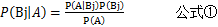此公式是表名给定元组数据A，其在A的条件下属于类别Bj的概率，由于A的每一列之间相互独立的，互不影响，因此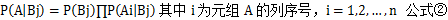在求出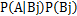之后，因为P(A)对每一个类别都相同，因此可通过对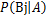的数值进行比较，因此就有：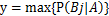得出概率最大的即为相应的预测类别。

在进行贝叶斯算法应用时候由于数据量较大而且较为复杂，因此经常会遇见概率为0的值，那么概率为0的值怎么办呐？有一个简单的技巧来避免该问题，可以假定训练数据库D很大，以至于对每个计数加1造成的估计概率的变化忽略不计，但可以方便的避免概率值为0.这种概率估计技术成为拉普拉斯校准或者拉普拉斯估计法。对元组中每一列属性的不重复的数据项计数加1,并且在分母计算上也加1，这样可以有效地避免概率为0的问题。

同时在运用python进行计算概率时候，我们可以通过取对数进行相应的计算，因为数据量过大的话会造成某项概率过小，但是我们按照数学的方法，y=x是单调递增，那么y=lnx也

是单调递增的这个思想，即x1>x2 那么lnx1>lnx2；通过对数之间的公式运算,如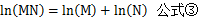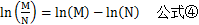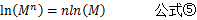朴素贝叶斯的python实现
# -*- coding: utf-8 -*-

from numpy import *
from functools import reduce

# 广告、垃圾标识
adClass = 1

def loadDataSet():
"""加载数据集合及其对应的分类"""
wordsList = [['周六', '公司', '一起', '聚餐', '时间'],
['优惠', '返利', '打折', '优惠', '金融', '理财'],
['喜欢', '机器学习', '一起', '研究', '欢迎', '贝叶斯', '算法', '公式'],
['公司', '发票', '税点', '优惠', '增值税', '打折'],
['北京', '今天', '雾霾', '不宜', '外出', '时间', '在家', '讨论', '学习'],
['招聘', '兼职', '日薪', '保险', '返利']]
# 1 是, 0 否
classVec = [0, 1, 0, 1, 0, 1]
return wordsList, classVec

# python中的& | 是位运算符   and or是逻辑运算符 当and的运算结果为true时候返回的并不是true而是运算结果最后一位变量的值
# 当and返回的结果是false时候，如果A AND B 返回的是第一个false的值，如果a为false 则返回a，如果a不是false，那么返回b
# 如果a or b 为true时候，返回的是第一个真的变量的值，如果a,b都为真时候那么返回a 如果a为假b为真那么返回b
# a & b a和b为两个set,返回结果取a和b的交集  a|b a和b为两个set,返回结果为两个集合的不重复并集
def doc2VecList(docList):
# 从第一个和第二个集合开始进行并集操作，最后返回一个不重复的并集
a = list(reduce(lambda x, y: set(x) | set(y), docList))
return a

def words2Vec(vecList, inputWords):
"""把单子转化为词向量"""
# 转化成以一维数组
resultVec =  * len(vecList)
for word in inputWords:
if word in vecList:
# 在单词出现的位置上的计数加1
resultVec[vecList.index(word)] += 1
else:
print('没有发现此单词')

return array(resultVec)

def trainNB(trainMatrix, trainClass):
"""计算，生成每个词对于类别上的概率"""
# 类别行数
numTrainClass = len(trainClass)
# 列数
numWords = len(trainMatrix)

# 全部都初始化为1， 防止出现概率为0的情况出现
# 见于韩家炜的数据挖掘概念与技术上的讲解，避免出现概率为0的状况，影响计算，因为在数量很大的情况下，在分子和分母同时+1的情况不会
# 影响主要的数据
p0Num = ones(numWords)
p1Num = ones(numWords)
# 相应的单词初始化为2
# 为了分子分母同时都加上某个数λ
p0Words = 2.0
p1Words = 2.0
# 统计每个分类的词的总数
# 训练数据集的行数作为遍历的条件，从1开始
# 如果当前类别为1，那么p1Num会加上当前单词矩阵行数据，依次遍历
# 如果当前类别为0，那么p0Num会加上当前单词矩阵行数据，依次遍历
# 同时统计当前类别下单词的个数和p1Words和p0Words
for i in range(numTrainClass):
if trainClass[i] == 1:
# 数组在对应的位置上相加
p1Num += trainMatrix[i]
p1Words += sum(trainMatrix[i])
else:
p0Num += trainMatrix[i]
p0Words += sum(trainMatrix[i])
# 计算每种类型里面， 每个单词出现的概率
# 朴素贝叶斯分类中，y=x是单调递增函数，y=ln(x)也是单调的递增的
# 如果x1>x2 那么ln(x1)>ln(x2)
# 在计算过程中，由于概率的值较小，所以我们就取对数进行比较，根据对数的特性
# ln(MN) = ln(M)+ln(N)
# ln(M/N) = ln(M)-ln(N)
# ln(M**n)= nln(M)
# 注：其中ln可替换为log的任意对数底
p0Vec = log(p0Num / p0Words)
p1Vec = log(p1Num / p1Words)
# 计算在类别中1出现的概率，0出现的概率可通过1-p得到
pClass1 = sum(trainClass) / float(numTrainClass)
return p0Vec, p1Vec, pClass1

def classifyNB(testVec, p0Vec, p1Vec, pClass1):
# 朴素贝叶斯分类, max(p0， p1)作为推断的分类
# y=x 是单调递增的， y=ln(x)也是单调递增的。 ， 如果x1 > x2, 那么ln(x1) > ln(x2)
# 因为概率的值太小了，所以我们可以取ln， 根据对数特性ln(ab) = lna + lnb， 可以简化计算
# sum是numpy的函数，testVec是一个数组向量，p1Vec是一个1的概率向量，通过矩阵之间的乘机
# 获得p(X1|Yj)*p(X2|Yj)*...*p(Xn|Yj)*p(Yj)
# 其中pClass1即为p(Yj)
# 此处计算出的p1是用对数表示，按照上面所说的，对数也是单调的，而贝叶斯分类主要是通过比较概率
# 出现的大小，不需要确切的概率数据，因此下述表述完全正确
p1 = sum(testVec * p1Vec) + log(pClass1)
p0 = sum(testVec * p0Vec) + log(1 - pClass1)
if p0 > p1:
return 0
return 1

def printClass(words, testClass):
if testClass == adClass:
print(words, '推测为：广告邮件')
else:
print(words, '推测为：正常邮件')

def tNB():
# 从训练数据集中提取出属性矩阵和分类数据
docList, classVec = loadDataSet()
# 生成包含所有单词的list
# 此处生成的单词向量是不重复的
allWordsVec = doc2VecList(docList)
# 构建词向量矩阵
# 计算docList数据集中每一行每个单词出现的次数，其中返回的trainMat是一个数组的数组
trainMat = list(map(lambda x: words2Vec(allWordsVec, x), docList))
# 训练计算每个词在分类上的概率, p0V:每个单词在非分类出现的概率， p1V:每个单词在是分类出现的概率
# 其中概率是以ln进行计算的
# pClass1为类别中是1的概率
p0V, p1V, pClass1 = trainNB(trainMat, classVec)
# 测试数据集
testWords = ['公司', '聚餐', '讨论', '贝叶斯']
# 转换成单词向量，32个单词构成的数组，如果此单词在数组中，数组的项值置1
testVec = words2Vec(allWordsVec, testWords)
# 通过将单词向量testVec代入，根据贝叶斯公式，比较各个类别的后验概率，判断当前数据的分类情况
testClass = classifyNB(testVec, p0V, p1V, pClass1)
# 打印出测试结果
printClass(testWords, testClass)

testWords = ['公司', '保险', '金融']
# 转换成单词向量，32个单词构成的数组，如果此单词在数组中，数组的项值置1
testVec = words2Vec(allWordsVec, testWords)
# 通过将单词向量testVec代入，根据贝叶斯公式，比较各个类别的后验概率，判断当前数据的分类情况
testClass = classifyNB(testVec, p0V, p1V, pClass1)
# 打印出测试结果
printClass(testWords, testClass)

if __name__ == '__main__':
tNB()



展开全文python 数据挖掘
• 本文主要讲述朴素贝叶斯分类算法并实现中文数据集的舆情分析案例，希望这篇文章对大家有所帮助，提供些思路。内容包括： 1.朴素贝叶斯数学原理知识 2.naive_bayes用法及简单案例 3.中文文本数据集预处理 4.朴素...
本文主要讲述朴素贝叶斯分类算法并实现中文数据集的舆情分析案例，希望这篇文章对大家有所帮助，提供些思路。内容包括：
1.朴素贝叶斯数学原理知识
2.naive_bayes用法及简单案例
3.中文文本数据集预处理
4.朴素贝叶斯中文文本舆情分析
本篇文章为基础性文章，希望对你有所帮助，如果文章中存在错误或不足之处，还请海涵。同时，推荐大家阅读我以前的文章了解基础知识。
一. 朴素贝叶斯数学原理知识
该基础知识部分引用文章"机器学习之朴素贝叶斯(NB)分类算法与Python实现"（https://blog.csdn.net/moxigandashu/article/details/71480251），也强烈推荐大家阅读博主moxigandashu的文章，写得很好。同时作者也结合概率论讲解，提升下自己较差的数学。
朴素贝叶斯（Naive Bayesian）是基于贝叶斯定理和特征条件独立假设的分类方法，它通过特征计算分类的概率，选取概率大的情况，是基于概率论的一种机器学习分类（监督学习）方法，被广泛应用于情感分类领域的分类器。
下面简单回顾下概率论知识：
1.什么是基于概率论的方法？
通过概率来衡量事件发生的可能性。概率论和统计学是两个相反的概念，统计学是抽取部分样本统计来估算总体情况，而概率论是通过总体情况来估计单个事件或部分事情的发生情况。概率论需要已知数据去预测未知的事件。
例如，我们看到天气乌云密布，电闪雷鸣并阵阵狂风，在这样的天气特征(F)下，我们推断下雨的概率比不下雨的概率大，也就是p(下雨)>p(不下雨)，所以认为待会儿会下雨，这个从经验上看对概率进行判断。而气象局通过多年长期积累的数据，经过计算，今天下雨的概率p(下雨)=85%、p(不下雨)=15%，同样的 p(下雨)>p(不下雨)，因此今天的天气预报肯定预报下雨。这是通过一定的方法计算概率从而对下雨事件进行判断。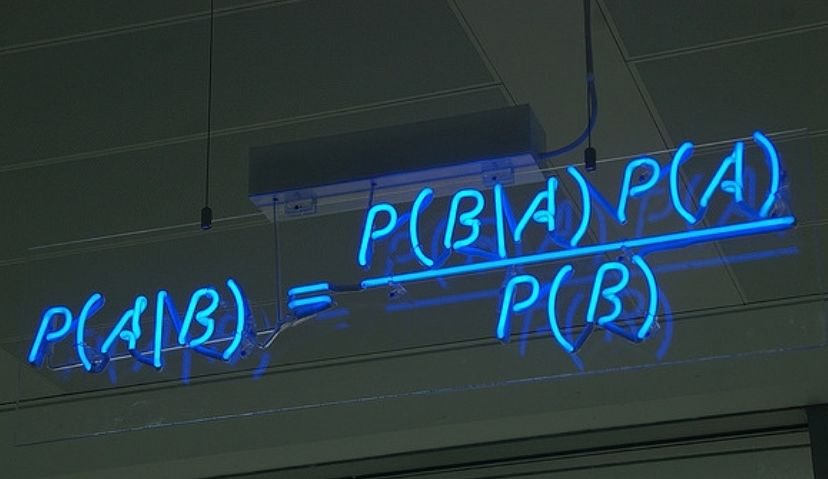2.条件概率
若Ω是全集，A、B是其中的事件（子集），P表示事件发生的概率，则条件概率表示某个事件发生时另一个事件发生的概率。假设事件B发生后事件A发生的概率为：设P(A)>0，则有 P(AB) = P(B|A)P(A) = P(A|B)P(B)。
设A、B、C为事件，且P(AB)>0，则有 P(ABC) = P(A)P(B|A)P(C|AB)。
现在A和B是两个相互独立的事件，其相交概率为 P(A∩B) = P(A)P(B)。
3.全概率公式
设Ω为试验E的样本空间，A为E的事件，B1、B2、…、Bn为Ω的一个划分，且P(Bi)>0，其中i=1,2,…,n，则：
P(A) = P(AB1)+P(AB2)+…+P(ABn)
    = P(A|B1)P(B1)+P(A|B2)P(B2)+...+P(A|Bn)P(Bn)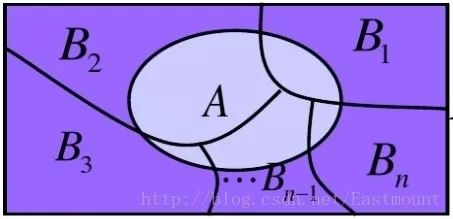全概率公式主要用途在于它可以将一个复杂的概率计算问题，分解为若干个简单事件的概率计算问题，最后应用概率的可加性求出最终结果。
示例：有一批同一型号的产品，已知其中由一厂生成的占30%，二厂生成的占50%，三长生成的占20%，又知这三个厂的产品次品概率分别为2%、1%、1%，问这批产品中任取一件是次品的概率是多少？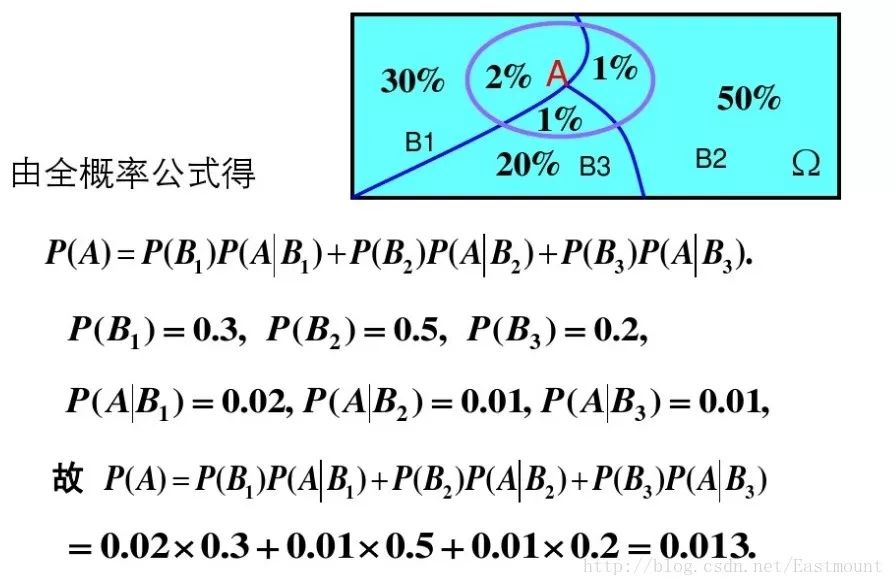参考百度文库资料：
https://wenku.baidu.com/view/05d0e30e856a561253d36fdb.html
4.贝叶斯公式
设Ω为试验E的样本空间，A为E的事件，如果有k个互斥且有穷个事件，即B1、B2、…、Bk为Ω的一个划分，且P(B1)+P(B2)+…+P(Bk)=1，P(Bi)>0（i=1,2,…,k)，则：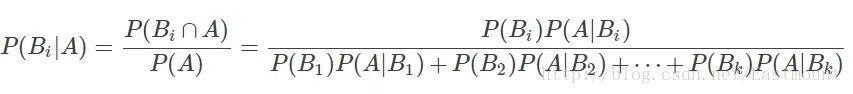P(A)：事件A发生的概率；
P(A∩B)：事件A和事件B同时发生的概率；
P(A|B)：事件A在时间B发生的条件下发生的概率；
意义：现在已知时间A确实已经发生，若要估计它是由原因Bi所导致的概率，则可用Bayes公式求出。
5.先验概率和后验概率
先验概率是由以往的数据分析得到的概率，泛指一类事物发生的概率，根据历史资料或主观判断未经证实所确定的概率。后验概率而是在得到信息之后再重新加以修正的概率，是某个特定条件下一个具体事物发生的概率。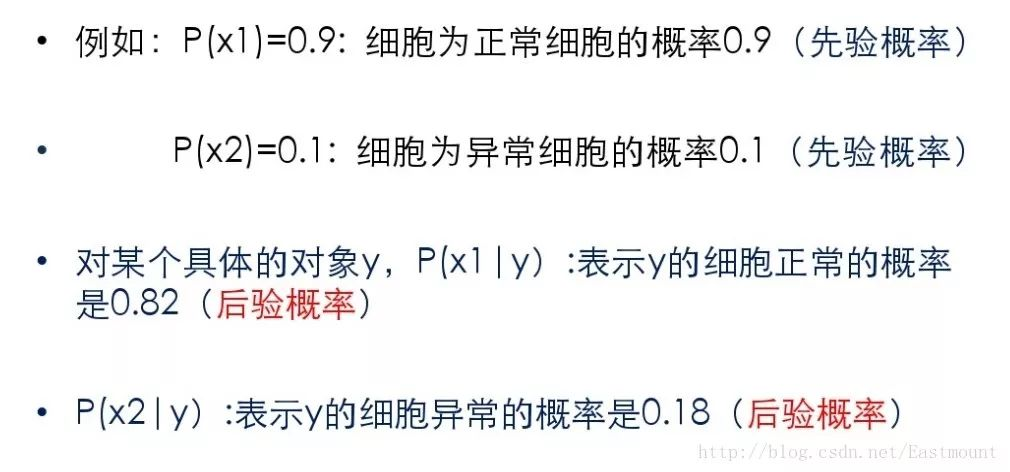6.朴素贝叶斯分类
贝叶斯分类器通过预测一个对象属于某个类别的概率，再预测其类别，是基于贝叶斯定理而构成出来的。在处理大规模数据集时，贝叶斯分类器表现出较高的分类准确性。
假设存在两种分类：

如果p1(x,y)>p2(x,y)，那么分入类别1

如果p1(x,y)<p2(x,y)，那么分入类别2

引入贝叶斯定理即为：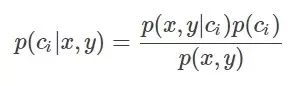其中，x、y表示特征变量，ci表示分类，p(ci|x,y)表示在特征为x,y的情况下分入类别ci的概率，因此，结合条件概率和贝叶斯定理有：

如果p(c1|x,y)>p(c2,|x,y)，那么分类应当属于类别c1

如果p(c1|x,y)<p(c2,|x,y)，那么分类应当属于类别c2

贝叶斯定理最大的好处是可以用已知的概率去计算未知的概率，而如果仅仅是为了比较p(ci|x,y)和p(cj|x,y)的大小，只需要已知两个概率即可，分母相同，比较p(x,y|ci)p(ci)和p(x,y|cj)p(cj)即可。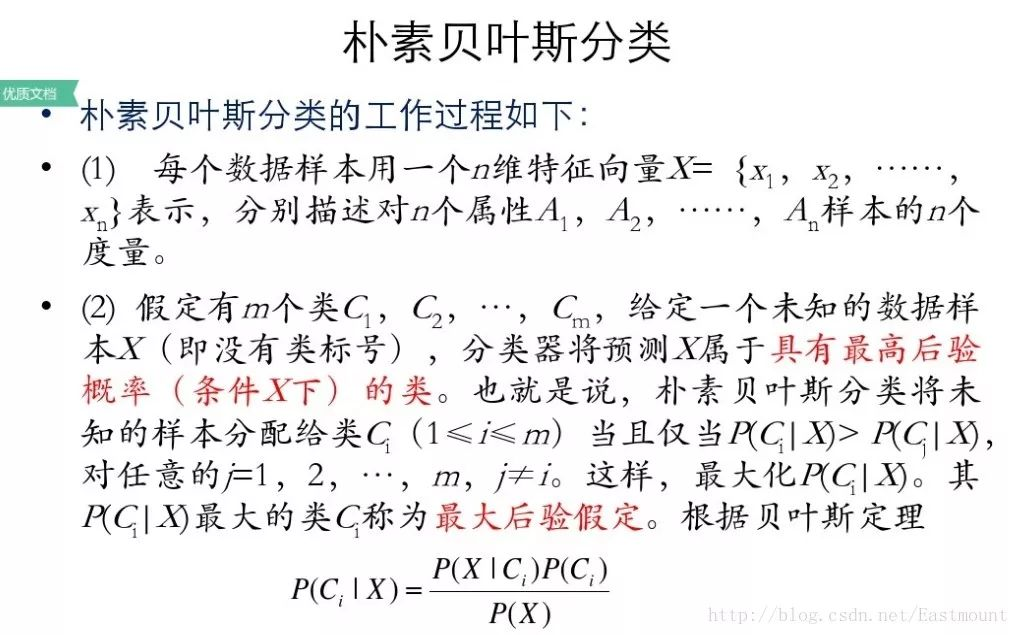7.示例讲解
假设存在14天的天气情况和是否能打网球，包括天气、气温、湿度、风等，现在给出新的一天天气情况，需要判断我们这一天可以打网球吗？首先统计出各种天气情况下打网球的概率，如下图所示。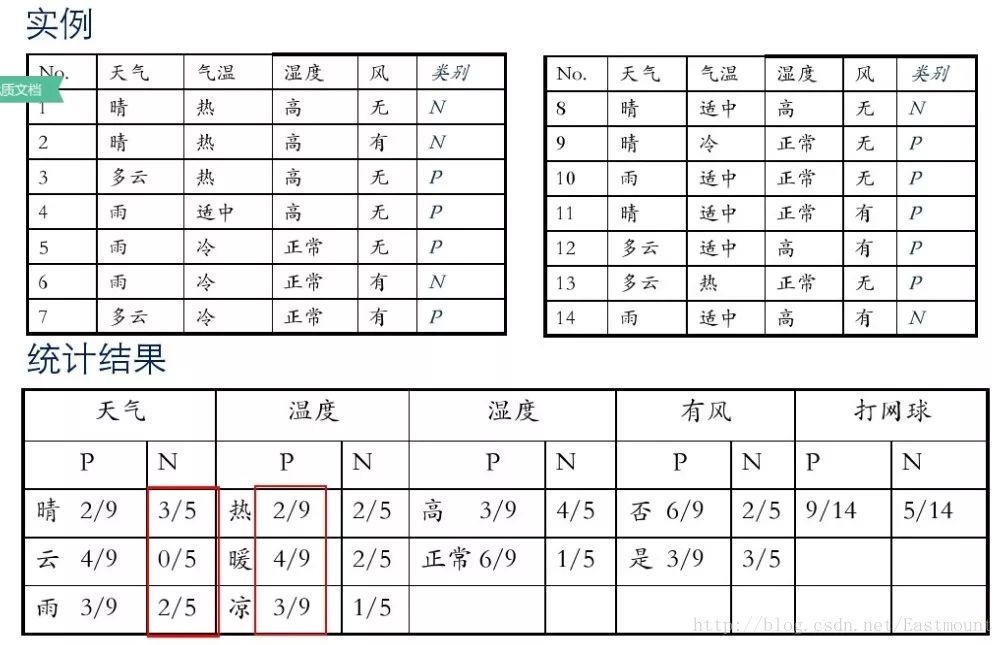接下来是分析过程，其中包括打网球yse和不打网球no的计算方法。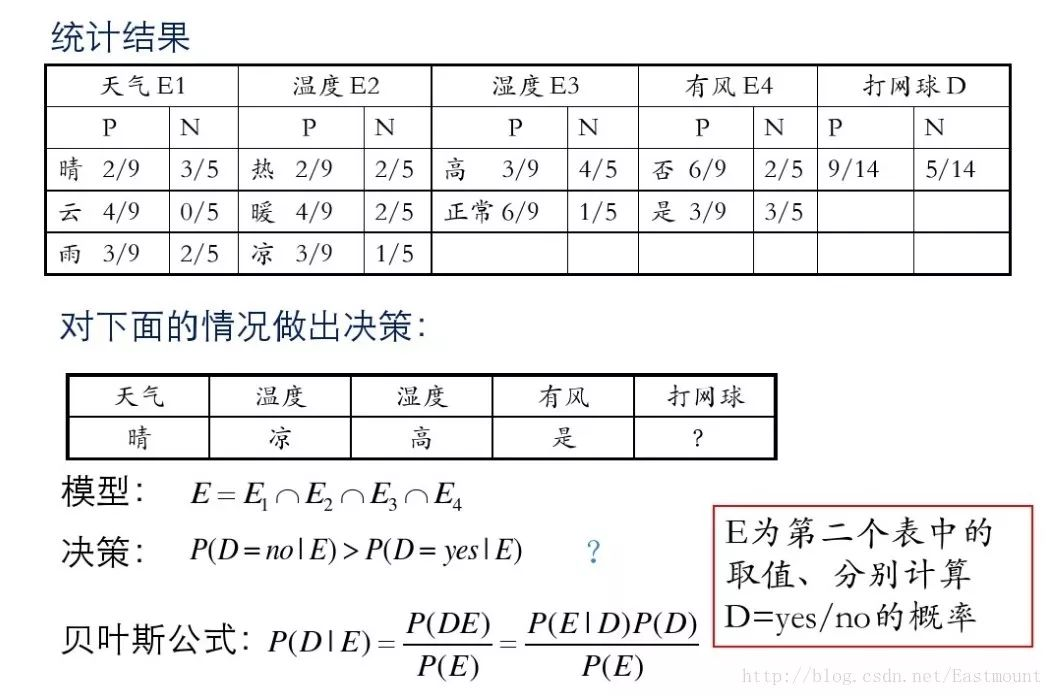最后计算结果如下，不去打网球概率为79.5%。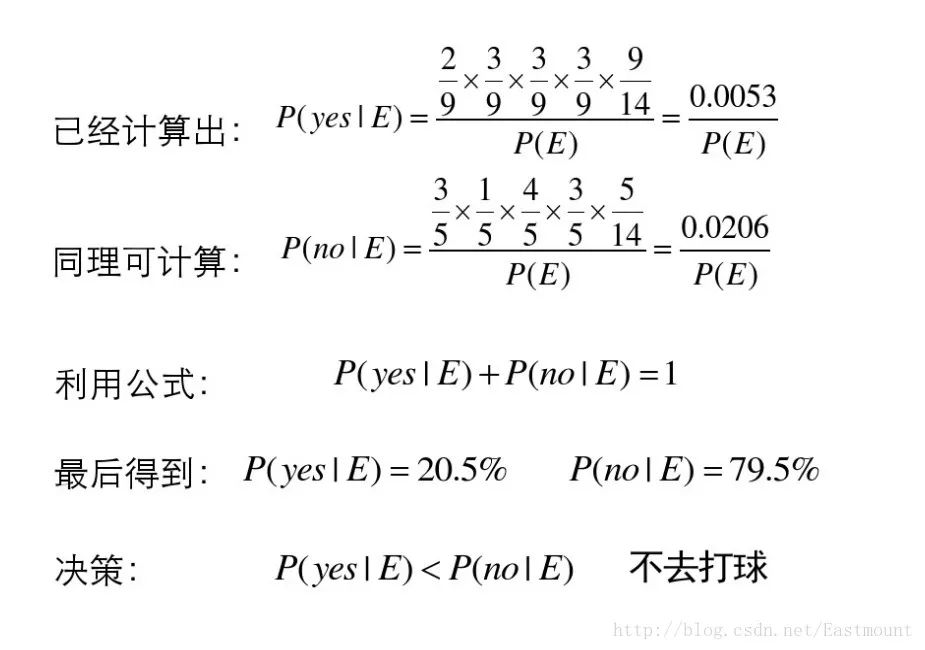8.优缺点
监督学习，需要确定分类的目标
对缺失数据不敏感，在数据较少的情况下依然可以使用该方法
可以处理多个类别 的分类问题
适用于标称型数据
对输入数据的形势比较敏感
由于用先验数据去预测分类，因此存在误差
二. naive_bayes用法及简单案例
scikit-learn机器学习包提供了3个朴素贝叶斯分类算法：
GaussianNB(高斯朴素贝叶斯)
MultinomialNB(多项式朴素贝叶斯)
BernoulliNB(伯努利朴素贝叶斯)
1.高斯朴素贝叶斯
调用方法为：sklearn.naive_bayes.GaussianNB(priors=None)。
下面随机生成六个坐标点，其中x坐标和y坐标同为正数时对应类标为2，x坐标和y坐标同为负数时对应类标为1。通过高斯朴素贝叶斯分类分析的代码如下：
1# -*- coding: utf-8 -*-
2import numpy as np
3from sklearn.naive_bayes import GaussianNB
4X = np.array([[-1, -1], [-2, -1], [-3, -2], [1, 1], [2, 1], [3, 2]])
5Y = np.array([1, 1, 1, 2, 2, 2])
6clf = GaussianNB()
7clf.fit(X, Y)
8pre = clf.predict(X)
9print u"数据集预测结果:", pre
10print clf.predict([[-0.8, -1]])
11
12clf_pf = GaussianNB()
13clf_pf.partial_fit(X, Y, np.unique(Y)) #增加一部分样本
14print clf_pf.predict([[-0.8, -1]])

输出如下图所示，可以看到[-0.8, -1]预测结果为1类，即x坐标和y坐标同为负数。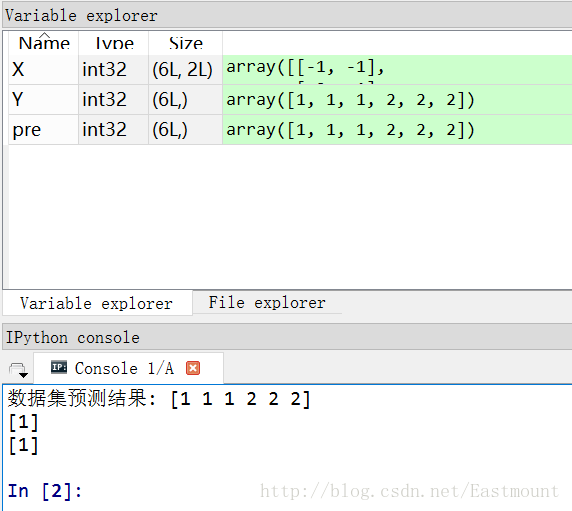2.多项式朴素贝叶斯
多项式朴素贝叶斯：sklearn.naive_bayes.MultinomialNB(alpha=1.0, fit_prior=True, class_prior=None)主要用于离散特征分类，例如文本分类单词统计，以出现的次数作为特征值。
参数说明：alpha为可选项，默认1.0，添加拉普拉修/Lidstone平滑参数；fit_prior默认True，表示是否学习先验概率，参数为False表示所有类标记具有相同的先验概率；class_prior类似数组，数组大小为(n_classes,)，默认None，类先验概率。
3.伯努利朴素贝叶斯
伯努利朴素贝叶斯：sklearn.naive_bayes.BernoulliNB(alpha=1.0, binarize=0.0, fit_prior=True,class_prior=None)。类似于多项式朴素贝叶斯，也主要用于离散特征分类，和MultinomialNB的区别是：MultinomialNB以出现的次数为特征值，BernoulliNB为二进制或布尔型特性
下面是朴素贝叶斯算法常见的属性和方法。

class_prior_属性

观察各类标记对应的先验概率，主要是class_prior_属性，返回数组。代码如下：
1print clf.class_prior_
2#[ 0.5  0.5]


class_count_属性

获取各类标记对应的训练样本数，代码如下：
1print clf.class_count_
2#[ 3.  3.]


theta_属性

获取各个类标记在各个特征上的均值，代码如下：
1print clf.theta_
2#[[-2.         -1.33333333]
3# [ 2.          1.33333333]]


sigma_属性

获取各个类标记在各个特征上的方差，代码如下：
1print clf.theta_
2#[[-2.         -1.33333333]
3# [ 2.          1.33333333]]


fit(X, y, sample_weight=None)

训练样本，X表示特征向量，y类标记，sample_weight表各样本权重数组。
1#设置样本不同的权重
2clf.fit(X,Y,np.array([0.05,0.05,0.1,0.1,0.1,0.2,0.2,0.2]))
3print clf
4print clf.theta_
5print clf.sigma_

输出结果如下所示：
1GaussianNB()
2[[-2.25 -1.5 ]
3 [ 2.25  1.5 ]]
4[[ 0.6875  0.25  ]
5 [ 0.6875  0.25  ]]


partial_fit(X, y, classes=None, sample_weight=None)

增量式训练，当训练数据集数据量非常大，不能一次性全部载入内存时，可以将数据集划分若干份，重复调用partial_fit在线学习模型参数，在第一次调用partial_fit函数时，必须制定classes参数，在随后的调用可以忽略。
1import numpy as np
2from sklearn.naive_bayes import GaussianNB
3X = np.array([[-1,-1], [-2,-2], [-3,-3], [-4,-4], [-5,-5],
4              [1,1], [2,2], [3,3]])
5y = np.array([1, 1, 1, 1, 1, 2, 2, 2])
6clf = GaussianNB()
7clf.partial_fit(X,y,classes=[1,2],
8                sample_weight=np.array([0.05,0.05,0.1,0.1,0.1,0.2,0.2,0.2]))
9print clf.class_prior_
10print clf.predict([[-6,-6],[4,5],[2,5]])
11print clf.predict_proba([[-6,-6],[4,5],[2,5]])

输出结果如下所示：
1[ 0.4  0.6]
2[1 2 2]
3[[  1.00000000e+00   4.21207358e-40]
4 [  1.12585521e-12   1.00000000e+00]
5 [  8.73474886e-11   1.00000000e+00]]

可以看到点[-6,-6]预测结果为1，[4,5]预测结果为2，[2,5]预测结果为2。同时，predict_proba(X)输出测试样本在各个类标记预测概率值。

score(X, y, sample_weight=None)

返回测试样本映射到指定类标记上的得分或准确率。
1pre = clf.predict([[-6,-6],[4,5],[2,5]])
2print clf.score([[-6,-6],[4,5],[2,5]],pre)
3#1.0

最后给出一个高斯朴素贝叶斯算法分析小麦数据集案例，代码如下：
1# -*- coding: utf-8 -*-
2#第一部分 载入数据集
3import pandas as pd
4X = pd.read_csv("seed_x.csv")
5Y = pd.read_csv("seed_y.csv")
6print X
7print Y
8
9#第二部分 导入模型
10from sklearn.naive_bayes import GaussianNB
11clf = GaussianNB()
12clf.fit(X, Y)
13pre = clf.predict(X)
14print u"数据集预测结果:", pre
15
16#第三部分 降维处理
17from sklearn.decomposition import PCA
18pca = PCA(n_components=2)
19newData = pca.fit_transform(X)
20print newData[:4]
21
22#第四部分 绘制图形
23import matplotlib.pyplot as plt
24L1 = [n for n in newData]
25L2 = [n for n in newData]
26plt.scatter(L1,L2,c=pre,s=200)
27plt.show()

输出如下图所示：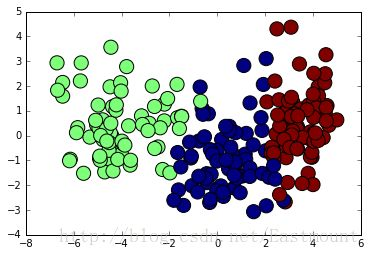最后对数据集进行评估，主要调用sklearn.metrics类中classification_report函数实现的，代码如下：
1from sklearn.metrics import classification_report
2print(classification_report(Y, pre))

运行结果如下所示，准确率、召回率和F特征为91%。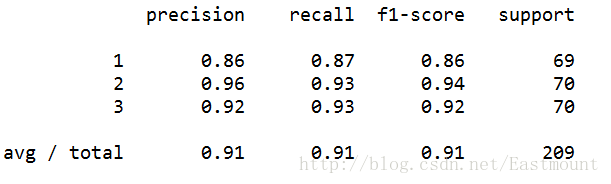补充下Sklearn机器学习包常用的扩展类。
1#监督学习
2sklearn.neighbors #近邻算法
3sklearn.svm #支持向量机
4sklearn.kernel_ridge #核-岭回归
5sklearn.discriminant_analysis #判别分析
6sklearn.linear_model #广义线性模型
7sklearn.ensemble #集成学习
8sklearn.tree #决策树
9sklearn.naive_bayes #朴素贝叶斯
10sklearn.cross_decomposition #交叉分解
11sklearn.gaussian_process #高斯过程
12sklearn.neural_network #神经网络
13sklearn.calibration #概率校准
14sklearn.isotonic #保守回归
15sklearn.feature_selection #特征选择
16sklearn.multiclass #多类多标签算法
17
18#无监督学习
19sklearn.decomposition #矩阵因子分解sklearn.cluster # 聚类
20sklearn.manifold # 流形学习
21sklearn.mixture # 高斯混合模型
22sklearn.neural_network # 无监督神经网络
23sklearn.covariance # 协方差估计
24
25#数据变换
26sklearn.feature_extraction # 特征提取sklearn.feature_selection # 特征选择
27sklearn.preprocessing # 预处理
28sklearn.random_projection # 随机投影
29sklearn.kernel_approximation # 核逼近

三. 中文文本数据集预处理
假设现在需要判断一封邮件是不是垃圾邮件，其步骤如下：
数据集拆分成单词，中文分词技术
计算句子中总共多少单词，确定词向量大小
句子中的单词转换成向量，BagofWordsVec
计算P(Ci)，P(Ci|w)=P(w|Ci)P(Ci)/P(w)，表示w特征出现时，该样本被分为Ci类的条件概率
判断P(w[i]C)和P(w[i]C)概率大小，两个集合中概率高的为分类类标
下面讲解一个具体的实例。
1.数据集读取
假设存在如下所示10条Python书籍订单评价信息，每条评价信息对应一个结果（好评和差评），如下图所示：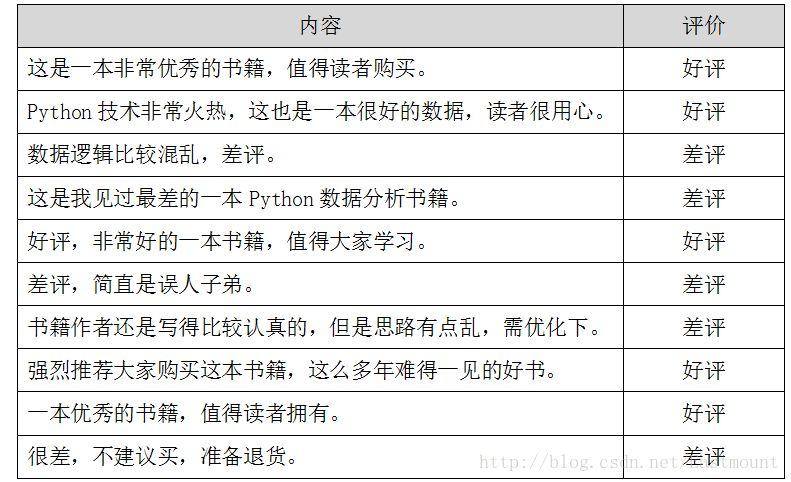数据存储至CSV文件中，如下图所示。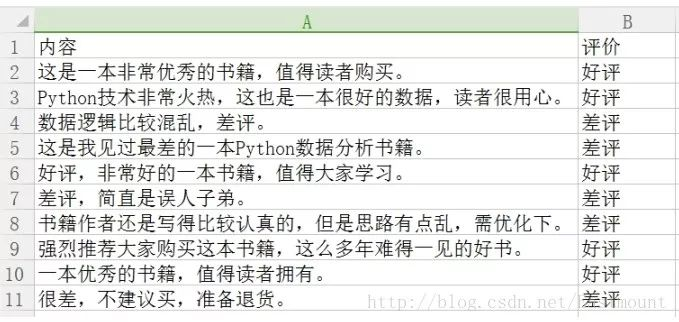下面采用pandas扩展包读取数据集。代码如下所示：
1# -*- coding: utf-8 -*-
2import numpy as np
3import pandas as pd
4
5data = pd.read_csv("data.csv",encoding='gbk')
6print data
7
8#取表中的第1列的所有值
9print u"获取第一列内容"
10col = data.iloc[:,0]
11#取表中所有值
12arrs = col.values
13for a in arrs:
14    print a

输出结果如下图所示，同时可以通过data.iloc[:,0]获取第一列的内容。
2.中文分词及过滤停用词
接下来作者采用jieba工具进行分词，并定义了停用词表，即：
stopwords = {}.fromkeys([’，’, ‘。’, ‘！’, ‘这’, ‘我’, ‘非常’])
完整代码如下所示：
1# -*- coding: utf-8 -*-
2import numpy as np
3import pandas as pd
4import jieba
5
6data = pd.read_csv("data.csv",encoding='gbk')
7print data
8
9#取表中的第1列的所有值
10print u"获取第一列内容"
11col = data.iloc[:,0]
12#取表中所有值
13arrs = col.values
14#去除停用词
15stopwords = {}.fromkeys(['，', '。', '！', '这', '我', '非常'])
16
17print u"\n中文分词后结果:"
18for a in arrs:
19    #print a
20    seglist = jieba.cut(a,cut_all=False)     #精确模式
21    final = ''
22    for seg in seglist:
23        seg = seg.encode('utf-8')
24        if seg not in stopwords: #不是停用词的保留
25            final += seg
26    seg_list = jieba.cut(final, cut_all=False)
27    output = ' '.join(list(seg_list))         #空格拼接
28    print output

然后分词后的数据如下所示，可以看到标点符号及“这”、“我”等词已经过滤。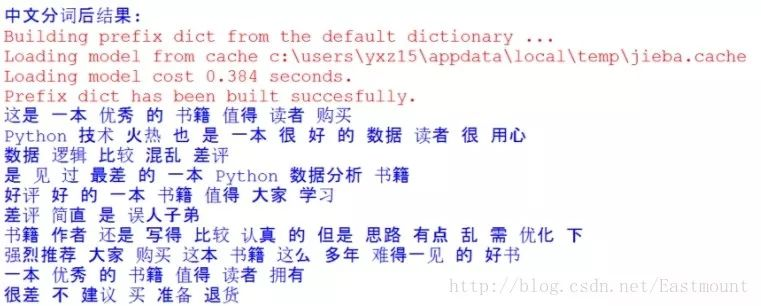3.词频统计
接下来需要将分词后的语句转换为向量的形式，这里使用CountVectorizer实现转换为词频。如果需要转换为TF-IDF值可以使用TfidfTransformer类。词频统计完整代码如下所示：
1# -*- coding: utf-8 -*-
2import numpy as np
3import pandas as pd
4import jieba
5
6data = pd.read_csv("data.csv",encoding='gbk')
7print data
8
9#取表中的第1列的所有值
10print u"获取第一列内容"
11col = data.iloc[:,0]
12#取表中所有值
13arrs = col.values
14#去除停用词
15stopwords = {}.fromkeys(['，', '。', '！', '这', '我', '非常'])
16
17print u"\n中文分词后结果:"
18corpus = []
19for a in arrs:
20    #print a
21    seglist = jieba.cut(a,cut_all=False)     #精确模式
22    final = ''
23    for seg in seglist:
24        seg = seg.encode('utf-8')
25        if seg not in stopwords: #不是停用词的保留
26            final += seg
27    seg_list = jieba.cut(final, cut_all=False)
28    output = ' '.join(list(seg_list))         #空格拼接
29    print output
30    corpus.append(output)
31
32#计算词频
33from sklearn.feature_extraction.text import CountVectorizer
34from sklearn.feature_extraction.text import TfidfTransformer
35
36vectorizer = CountVectorizer() #将文本中的词语转换为词频矩阵
37X = vectorizer.fit_transform(corpus) #计算个词语出现的次数
38word = vectorizer.get_feature_names() #获取词袋中所有文本关键词
39for w in word: #查看词频结果
40    print w,
41print ''
42print X.toarray()

输出结果如下所示，包括特征词及对应的10行数据的向量，这就将中文文本数据集转换为了数学向量的形式，接下来就是对应的数据分析了。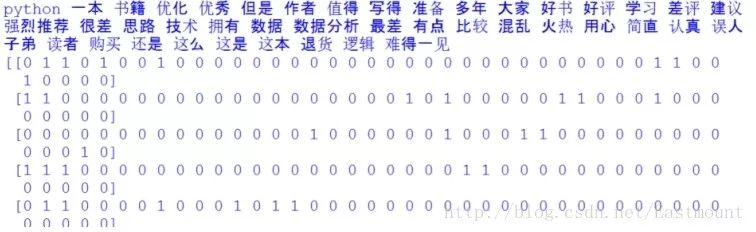如下所示得到一个词频矩阵，每行数据集对应一个分类类标，可以预测新的文档属于哪一类。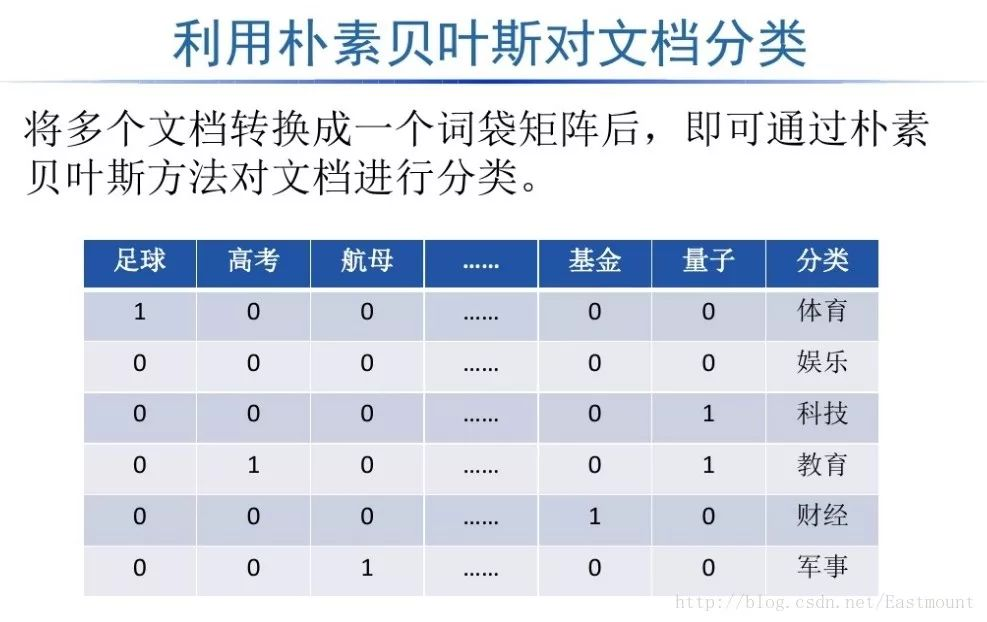TF-IDF相关知识推荐我的文章： [python] 使用scikit-learn工具计算文本TF-IDF值（https://blog.csdn.net/eastmount/article/details/50323063）
四. 朴素贝叶斯中文文本舆情分析
最后给出朴素贝叶斯分类算法分析中文文本数据集的完整代码。
1# -*- coding: utf-8 -*-
2import numpy as np
3import pandas as pd
4import jieba
5
6#http://blog.csdn.net/eastmount/article/details/50323063
7#http://blog.csdn.net/eastmount/article/details/50256163
8#http://blog.csdn.net/lsldd/article/details/41542107
9
10####################################
11#         第一步 读取数据及分词
12#
13data = pd.read_csv("data.csv",encoding='gbk')
14print data
15
16#取表中的第1列的所有值
17print u"获取第一列内容"
18col = data.iloc[:,0]
19#取表中所有值
20arrs = col.values
21
22#去除停用词
23stopwords = {}.fromkeys(['，', '。', '！', '这', '我', '非常'])
24
25print u"\n中文分词后结果:"
26corpus = []
27for a in arrs:
28    #print a
29    seglist = jieba.cut(a,cut_all=False)     #精确模式
30    final = ''
31    for seg in seglist:
32        seg = seg.encode('utf-8')
33        if seg not in stopwords: #不是停用词的保留
34            final += seg
35    seg_list = jieba.cut(final, cut_all=False)
36    output = ' '.join(list(seg_list))         #空格拼接
37    print output
38    corpus.append(output)
39
40####################################
41#         第二步 计算词频
42#
43from sklearn.feature_extraction.text import CountVectorizer
44from sklearn.feature_extraction.text import TfidfTransformer
45
46vectorizer = CountVectorizer() #将文本中的词语转换为词频矩阵
47X = vectorizer.fit_transform(corpus) #计算个词语出现的次数
48word = vectorizer.get_feature_names() #获取词袋中所有文本关键词
49for w in word: #查看词频结果
50    print w,
51print ''
52print X.toarray()
53
54
55####################################
56#         第三步 数据分析
57#
58from sklearn.naive_bayes import MultinomialNB
59from sklearn.metrics import precision_recall_curve
60from sklearn.metrics import classification_report
61
62#使用前8行数据集进行训练，最后两行数据集用于预测
63print u"\n\n数据分析:"
64X = X.toarray()
65x_train = X[:8]
66x_test = X[8:]
67#1表示好评 0表示差评
68y_train = [1,1,0,0,1,0,0,1]
69y_test = [1,0]
70
71#调用MultinomialNB分类器
72clf = MultinomialNB().fit(x_train, y_train)
73pre = clf.predict(x_test)
74print u"预测结果:",pre
75print u"真实结果:",y_test
76
77from sklearn.metrics import classification_report
78print(classification_report(y_test, pre))

输出结果如下所示，可以看到预测的两个值都是正确的。即“一本优秀的书籍，值得读者拥有。”预测结果为好评（类标1），“很差，不建议买，准备退货。”结果为差评（类标0）。
1数据分析:
2预测结果: [1 0]
3真实结果: [1, 0]
4             precision    recall  f1-score   support
5
6          0       1.00      1.00      1.00         1
7          1       1.00      1.00      1.00         1
8
9avg / total       1.00      1.00      1.00         2

但存在一个问题，由于数据量较小不具备代表性，而真实分析中会使用海量数据进行舆情分析，预测结果肯定页不是100%的正确，但是需要让实验结果尽可能的好。最后补充一段降维绘制图形的代码，如下：
1#降维绘制图形
2from sklearn.decomposition import PCA
3pca = PCA(n_components=2)
4newData = pca.fit_transform(X)
5print newData
6
7pre = clf.predict(X)
8Y = [1,1,0,0,1,0,0,1,1,0]
9import matplotlib.pyplot as plt
10L1 = [n for n in newData]
11L2 = [n for n in newData]
12plt.scatter(L1,L2,c=pre,s=200)
13plt.show()

输出结果如图所示，预测结果和真实结果都是一样的，即[1,1,0,0,1,0,0,1,1,0]。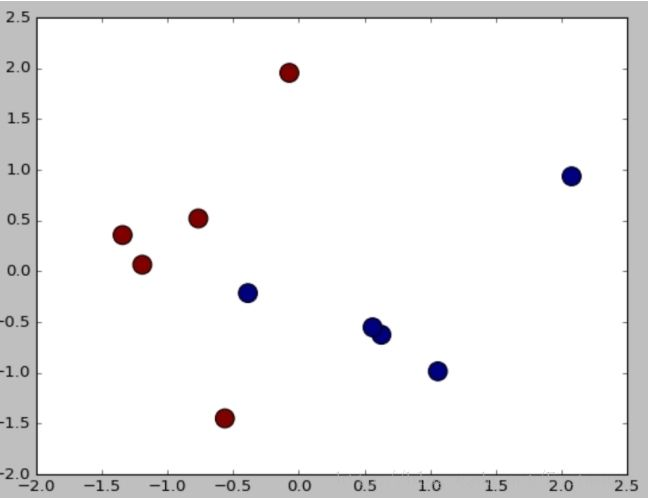展开全文大数据 数据挖掘 机器学习
• P(X=x|Y=ck)=P(X=x1,X=2,...,X=xi|Y=ck)=P(X=x1,X=2,...,X=xi)/P(Y=ck) 因为朴素贝叶斯对条件概率做了独立性假设，所以P(X=x1,X=x2,...,X=xi)/P(Y=ck)=P(X=x1)P(X=x2)...P(X=xi)/P(Y=ck)= ∏P(X=xi|Y=ck) 将上式代...文章来源：公众号-俊红的数据分析之路一、统计知识01|随机事件：1、概念随机事件是在随机试验中，可能出现也可能不出现，而在大量重复试验中具有某种规律性的事件叫做随机事件(简称事件)。随机事件通常用大写英文字母A、B、C等表示。随机试验中的每一个可能出现的试验结果称为这个试验的一个样本点，记作ωi。全体样本点组成的集合称为这个试验的样本空间，记作Ω．即Ω={ω1，ω2，…，ωn，…}随机事件中的事件形式可能由各种形式，比如{"正面","反面"}，{"优","良","差"}。2、条件概率P(A|B)=P(AB)/P(B)表示在事件B发生的情况下事件A发生的概率。3、一些性质概率的有限可加性：若事件A1、A2、......、Ai.....、Aj这些事件两两互斥，则P(∑Ai)=∑P(Ai),表示所有事件发生的概率等于各个事件发生的概率之和。概率的乘法公式：P(AB)=P(A)P(B|A)=P(B)P(A|B)。若事件A与B相互独立，则P(AB)=P(A)P(B),推广到有限多个事件时可表示为：P(A1A2A3.....An)=P(A1)P(A2|A1)P(A3|A1A2)......P(An|A1A2......An-1)。4、全概率公式对于比较复杂的概率事件的计算，经常会把它分解成若干个简单事件的和，通过分别计算这些简单事件的概率，然后利用概率的可加性计算出所求事件的概率。假设事件A1、A2、......、An是Ω的一个划分，即两两互斥，且∑Ai=Ω，则B=B∑Ai，再由概率的有限可加性得，P(B)=P(B∑Ai)=P(∑BAi)。再由概率得乘法公式得P(B)=P(∑BAi)=∑P(Ai)P(B|Ai),这就是全概率公式。5、贝叶斯公式假设事件A1、A2、......、An是Ω的一个划分，B=B∑Ai=∑BAi，则B发生条件下Ai发生得概率为：P(Ai|B)=P(AiB)/P(B)。由乘法公式和全概率公式得P(Ai|B)=P(Ai)P(B|Ai)/∑P(Ai)P(B|Ai)。该公式就是贝叶斯公式。02|一维随机变量：1、概念一般意义上概率是针对于某一随机事件而言得，为更深入得研究随机试验得结果，我们引入随机变量得概念，随机变量得基本思想是把随机试验的结果数量化，从而可用一个变量去描述随机事件。对于随机事件中出现的某一事件我们用变量的形式去表示。比如{"正面","反面"}可以表示为{1,0},{"优","良","差"}可以表示为{1，2，3}。2、随机变量分布假设X是一随机变量，他可能取值为X1,X2,....,Xk,并且取各个值对应的概率分别为P1,P2,......Pk,即P(X=Xk)=Pk,K=1,2,....,该式称为随机变量X的概率分布。03|多维随机变量：1、概念通过随机变量来描述某一随机事件时，有时候仅用一个维度上的变量去表示是不够的，比如描述平面的某一个点的位置就需要用X,Y两条轴（即两个维度）去表示才可以，如果是描述空间中某个点可能需要X,Y,Z三个维度去表示才可以，我们把一个随机变量需要从n个维度去表示的变量称为n维随机变量。一般地，当n=2时，称(X,Y)为二维随机变量，二维随机变量的联合分布为：F(x,y)=P(X≤x,Y≤y)，其中P(X≤x,Y≤y)表示随机事件{X≤x}，{Y≤y}同时发生的概率。若事件X与Y相互独立，则P(X≤x,Y≤y)=P(X≤x)P(Y≤y)。条件分布：P(X≤x|Y≤y)=P(X≤x,Y≤y)/P(Y≤y)。二、算法知识01|原理：朴素贝叶斯算法就是根据贝叶斯公式来对未知事物进行分类，通过已知条件(X=x)计算未知事物分别属于各个类别(Y=ck)时对应的概率，然后把未知事物判别为概率最大的那一类。贝叶斯公式：P(Y=ck|X=x)=P(Y=ck)P(X=x|Y=ck)/∑P(Y=ck)P(X=x|Y=ck)。02|学习过程：我们要想计算出未知事物属于哪一个类别的概率较大，即P(Y=ck|X=x)在x等于多少时概率最大，需要先算出P(Y=ck)和P(X=x|Y=ck)。这两个值可以通过训练集求得。在具体的求取过程中需要用到一种估计方法就是极大似然估计。极大似然估计是一种概率论在统计中的应用，说的是已知某个随机样本满足某种概率分布，但是其中具体的参数不清楚，参数估计就是通过若干次试验，观察其结果，利用结果推出参数的大概值，一般把这个结果作为估计值。在这里我们用极大似然估计的方法来给P(Y=ck)以及P(X=x|Y=ck)估值，把训练集中的所有值当成是若干次试验以后得到的结果，利用极大似然估计的思想，则P(Y=ck)=该类别的频数/集合总数。P(Y=ck)=∑I(Y=ck)/N，k=1,2,...,K, N为集合总数。P(X=x|Y=ck)=ck类下满足条件x的值的频数/属于ck类值的总数。P(X=x|Y=ck)=∑I(X=x,Y=ck)/∑I(Y=ck)，k=1,2,....,K上面的I为指示函数，是定义在某一集合X上的函数，表示其中有哪些元素属于某一子集A，常用于次数的统计，即满足某一条件的个数。预估出P(Y=ck)和P(X=x|Y=ck)的值以后，我们就可以利用贝叶斯公式对在X=x的条件下P(Y=ck|X=x)对应的分类是哪一类。上面的学习过程都只是针对于一维变量进行求取的，在实际的算法应用中，往往是多维的，即多个特征，于是乎：P(X=x|Y=ck)=P(X=x1,X=x2,...,X=xi|Y=ck)，xi表示变量x的第i个特征。P(X=x|Y=ck)=P(X=x1,X=2,...,X=xi|Y=ck)=P(X=x1,X=2,...,X=xi)/P(Y=ck)因为朴素贝叶斯对条件概率做了独立性假设，所以P(X=x1,X=x2,...,X=xi)/P(Y=ck)=P(X=x1)P(X=x2)...P(X=xi)/P(Y=ck)=∏P(X=xi|Y=ck)将上式代入贝叶斯公式中可得出：P(Y=ck|X=x)=P(Y=ck)∏P(X=xi|Y=ck)/∑P(Y=ck)∏P(X=xi|Y=ck)，因为分母∑P(Y=ck)∏P(X=xi|Y=ck)对每个分类ck来说，其值都是相等的，而我们最后是要比较每个ck对应的P(Y=ck|X=x)的大小，所以可以把分母去掉，最后学习到的模型为：y=f(x)=P(Y=ck)∏P(X=xi|Y=ck)。（其中∏P(X=xi|Y=ck)需要让i取不同的值(即不同的特征)然后用极大似然估计去估计。）03|测试过程：将测试数据集代入到学到的模型y=f(x)=P(Y=ck)∏P(X=xi|Y=ck)中，然后看不同分类所对应的概率，选择概率最大的分类为待测数据的分类。然后判断其预测的准确性。04|贝叶斯估计：在前面我们估计P(Y=ck)和P(X=x|Y=ck)的值的时候用的是极大似然估计，使用这种方法很有可能出现估计值为0的情况，因为有些特征可能不存在，所以其概率为0，但是P(X=x|Y=ck)=∏P(X=xi|Y=ck)，只要有一个特征为0，整个P(X=x|Y=ck)就会为0，这将影响到后续的概率计算，所以这种估计方法有的时候是行不通的，我们需要换另外的估计方法，就是贝叶斯估计。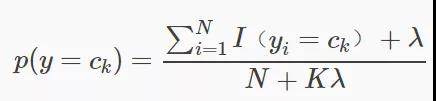K为类的个数。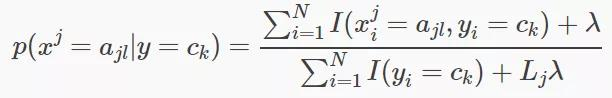Lj是第j维特征的最大取值。贝叶斯估计是在极大似然估计的基础上给分子分母分别加一个常数，当λ=1时称为拉普拉斯平滑。05|利用python对留言性质进行判断：本实例以及代码均来自于书籍《机器学习实战》第4章。1、背景某社区为了屏蔽掉一些侮辱性留言，需要构建一个快速过滤器，只要某条留言使用了负面或者侮辱性的语言，那么就将该留言标识为内容不当。2、准备数据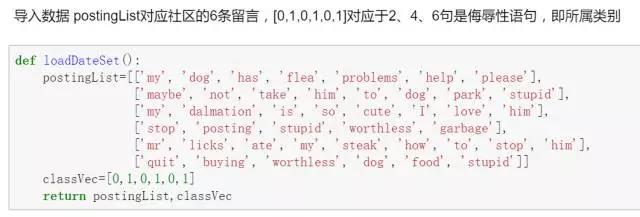3、数据处理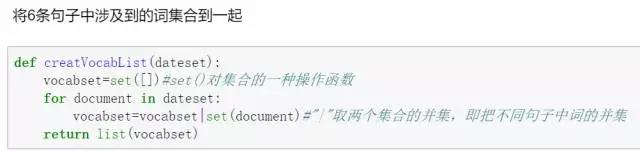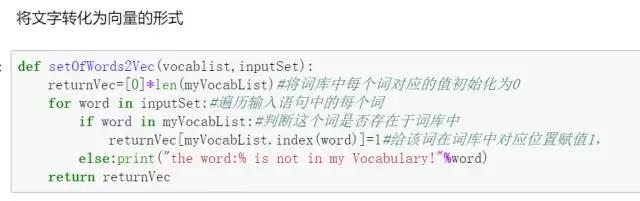4、计算先验概率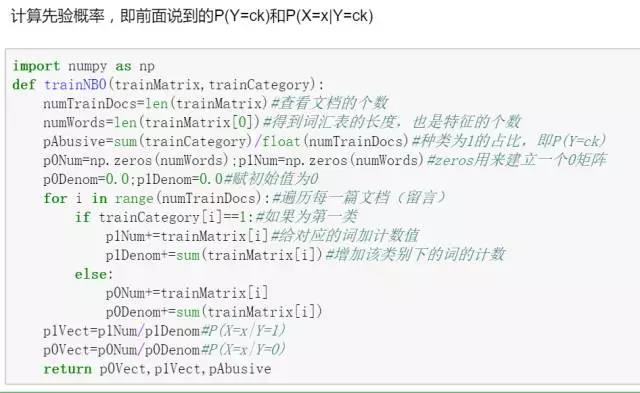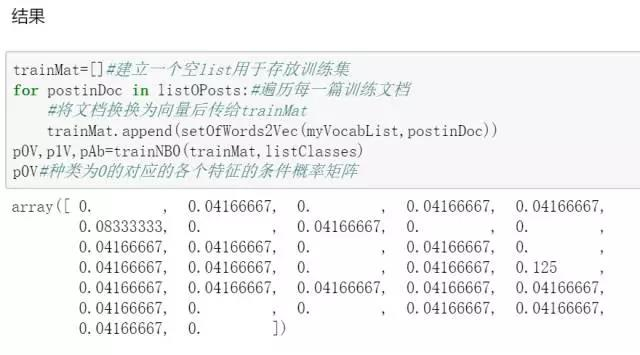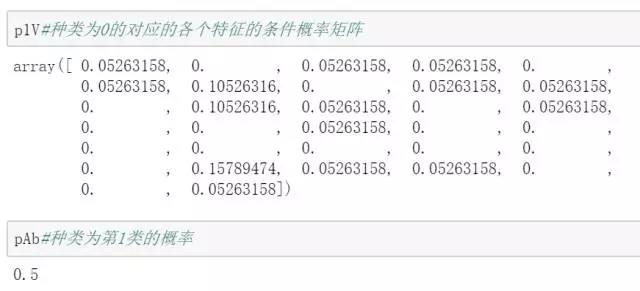5、调试算法在前面我们讲过采用极大似然估计中可能会出现概率为0的情况，在上面的结果代码中我们也可以看出，确实有一些结果为0的概率，为了避免这种情况，我们将采用拉普拉斯平滑。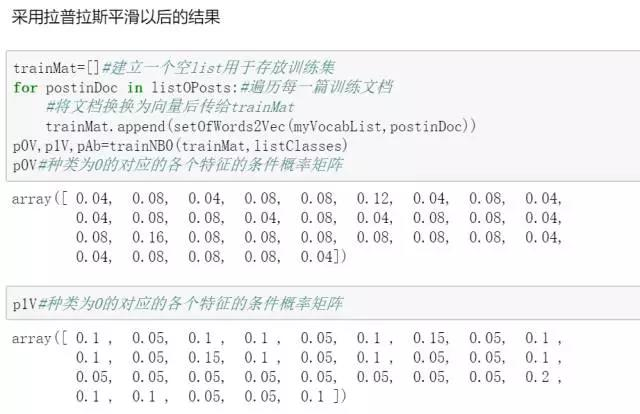6、应用算法最后就可利用模型y=f(x)=P(Y=ck)∏P(X=xi|Y=ck)对具体某一条留言的是否包含侮辱性评论进行判断了。
展开全文• 这正是朴素的含义，虽然朴素贝叶斯的分类效果不错，但是属性之间毕竟是有关联的，某个属性依赖于另外的属性，于是就有了半朴素贝叶斯分类器。 确认依赖 1.SOPDE方法。这种方法是假定所有的属性都依赖...
• 贝叶斯定理是统计学中非常重要的一个定理，以贝叶斯定理为基础的统计学派在统计学世界里占据着重要的地位，和概率学派从事件的随机性出发不同，贝叶斯统计学更多地是从观察者的角度出发，事件的随机性不过是观察者...
• 文章目录朴素贝叶斯法的学习和分类基本方法后验概率最大化的道理朴素贝叶斯法的参数估计极大似然估计贝叶斯估计补充知识 本笔记主要思路和内容源自《统计学习方法》，笔者采取了更易于理解的方式进行解读 朴素贝叶斯...机器学习 算法 统计学 统计模型
• 在开始学习具体的贝叶斯参数前，你可以先看看：朴素贝叶斯详解​mp.weixin.qq.com朴素贝叶斯一共有三种方法，分别是高斯朴素贝叶斯、多项式分布贝叶斯、伯努利朴素贝叶斯，在介绍不同方法的具体参数前，我们先看看这...
• 　朴素贝叶斯算法（Naive Bayes）是机器学习中常见的基本算法之一，主要用来做分类任务的。它是基于贝叶斯定理与条件独立性假设的分类方法。对于给定的训练数据集，首先基于特征条件独立性假设学习输入/输出的联合...机器学习
•机器学习 数据挖掘 算法
• 主要介绍了PHP实现机器学习之朴素贝叶斯算法,结合实例形式详细分析了朴素贝叶斯算法的概念、原理及php实现技巧,需要的朋友可以参考下
• ## 机器学习之朴素贝叶斯算法详解

万次阅读 多人点赞 2018-07-05 19:39:13
朴素贝叶斯公式： P(A|B)=P(A)P(B|A)P(B)P(A|B)=P(A)P(B|A)P(B) P(A|B) = \frac{P(A)P(B|A)}{P(B)} 一、概率基础知识： 条件概率是指事件A在另外一个事件B已经发生条件下的发生概率。 条件概率表示为： P(A|B)...机器学习
• 朴素贝叶斯原理详解1.知识准备2.贝叶斯定理3.贝叶斯定理在分类中的应用3.1条件独立3.2特征取离散值的条件概率3.3特征取连续值的条件概率高斯贝叶斯分类器:多项式贝叶斯分类器:伯努利贝叶斯分类器:4.条件概率的m估计5...机器学习 算法
• 一：贝叶斯定理 P(A∣B)P(A|B)P(A∣B) = P(A)P(B∣A)P(B)\frac{P(A)P(B|A)}{P(B)}P(B)P(A)P(B∣A)​，其中P(A∣B)P(A|B)P(A∣B)是在BBB发生条件下AAA发生的可能性。 二：特征条件独立性 如果AAA、BBB两事件相互独立...python Bayes
• 文章目录朴素贝叶斯朴素贝叶斯学习目标朴素贝叶斯引入朴素贝叶斯详解朴素贝叶斯构造朴素贝叶斯基本公式朴素贝叶斯参数估计特征值为离散值特征值为稀疏的离散值特征值为连续值三种不同的朴素贝叶斯多项式朴素贝叶斯...
• ## 详解朴素贝叶斯分类算法

万次阅读 多人点赞 2018-08-15 22:25:31
带你搞懂朴素贝叶斯分类算法   带你搞懂朴素贝叶斯分类算 贝叶斯分类是一类分类算法的总称，这类算法均以贝叶斯定理为基础，故统称为贝叶斯分类。而朴素朴素贝叶斯分类是贝叶斯分类中最简单，也是常见的一种分类...
• 本文实例讲述了Python实现朴素贝叶斯分类器的方法。分享给大家供大家参考，具体如下：贝叶斯定理贝叶斯定理是通过对观测值概率分布的主观判断(即先验概率)进行修正的定理，在概率论中具有重要地位。先验概率分布...
• 本文实例讲述了PHP实现机器学习之朴素贝叶斯算法。，具体如下：机器学习已经在我们的生活中变得随处可见了。比如从你在家的时候温控器开始工作到智能汽车以及我们口袋中...在这篇文章我将会使用朴素贝叶斯算法Clasif...
• 参考链接： Python朴素贝叶斯分类器 本文实例讲述了Python实现朴素贝叶斯分类器的方法。分享给大家供大家参考，具体如下： 贝叶斯定理 贝叶斯定理是通过对观测值概率分布的主观判断(即先验概率)进行修正的定理，...
• 文章首发于 朴素贝叶斯分类器详解 | 隐舍 分类问题定义 已知集合：C={y1,y2,...,yn}C=\{y_1, y_2, ..., y_n\}C={y1​,y2​,...,yn​}和I={x1,x2,...,xm}I=\{x_1, x_2, ..., x_m\}I={x1​,x2​,...,xm​}，确定映射...机器学习 人工智能 算法 深度学习...